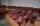# Trucks

Three lorries droved bricks. One drove n bricks at once, second m less bricks than the first and third 300 bricks more the first lorry. The first lorry went 4 times a day the largest went 3 times a day and the smallest 5 times a day.

How many bricks brought:

a / all lorries in one day.
b / all lorries per week / Sunday don't worked /?

Result

a = (Correct answer is: 12n-5m+900)b = (Correct answer is: 72n-30m+5400)#### Solution:Leave us a comment of example and its solution (i.e. if it is still somewhat unclear...):

Showing 0 comments:Be the first to comment!## Next similar examples:

1. CardsSuppose that are three cards in the hats. One is red on both sides, one of which is black on both sides, and a third one side red and the second black. We are pulled out of a hat randomly one card and we see that one side of it is red. What is the probabi
2. Holidays - on poolChildren's tickets to the swimming pool stands x € for an adult is € 2 more expensive. There was m children in the swimming pool and adults three times less. How many euros make treasurer for pool entry?
3. Theorem proveWe want to prove the sentense: If the natural number n is divisible by six, then n is divisible by three. From what assumption we started?
4. CandiesIn the box are 12 candies that look the same. Three of them are filled with nougat, five by nuts, four by cream. At least how many candies must Ivan choose to satisfy itself that the selection of two with the same filling? ?
5. Expressions 3If k(x+6)= 4x2 + 20, what is k(10)=?
6. Expression with powersIf x-1/x=5, find the value of x4+1/x4
7. Functions f,gFind g(1) if g(x) = 3x - x2 Find f(5) if f(x) = x + 1/2
8. CoefficientDetermine the coefficient of this sequence: 7.2; 2.4; 0.8
9. AlgebraX+y=5, find xy (find the product of x and y if x+y = 5)
10. SequenceWrite the first 6 members of these sequence: a1 = 5 a2 = 7 an+2 = an+1 +2 an
11. Quadratic function 2Which of the points belong function f:y= 2x2- 3x + 1 : A(-2, 15) B (3,10) C (1,4)
12. ChairsDetermine the number of seats in the seventh row and ninth row, if 3rd row has 14 seats and in every next row of seats has five more than the previous row.
13. BlocksThere are 9 interactive basic building blocks of an organization. How many two-blocks combinations are there?
14. ChordsHow many 4-tones chords (chord = at the same time sounding different tones) is possible to play within 7 tones?
15. AsymptoteWhat is the vertical asymptote of ?
16. GP membersThe geometric sequence has 10 members. The last two members are 2 and -1. Which member is -1/16?
17. Elimination methodSolve system of linear equations by elimination method: 5/2x + 3/5y= 4/15 1/2x + 2/5y= 2/15